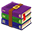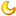# 基于51单片机的脉搏体温血压检测仪Proteus仿真程序73 |0 | 2020-1-23 01:01:11 | 显示全部楼层 |阅读模式
 以单片机为控制核心，设计一种电子血压计，同时具有测量体温的功能。该系统利用压力传感器采集压力信号，通过温度传感器采集温度信号，整个系统包括处理模块、测量模块、信号处理模块、显示模块以及电源处理模块；血压和体温通过按键进行切换。 基本要求： 1、用Proteus完成整体硬件设计原理图； 2、基于Keil C51编写软件，编译出所需的实际程序； 3、在Proteus硬件原理图中通过仿真验证的方式确定该电子血压计的可操作性。基于51单片机的电子血压计设计.zip (1.01 MB, 售价: 5 工控币) 2020-1-23 00:59 上传 点击文件名下载附件#include #include #include #define uint            unsigned int #define uchar           unsigned char #define ulong           unsigned long         //宏定义 #define LCD_DATA        P0                                 //定义P0口为LCD_DATA unsigned char getdata; //获取ADC转换回来的值 unsigned char dispbuf; unsigned int OverCounter = 0; unsigned char ptemp; bit OverFlg = 0; unsigned int temp,ppress = 0; float  press;         char KEY_TIMES = 0; char KEY_Set_TIMES = 0; sbit LCD_RS =P2^5; sbit LCD_RW =P2^6; sbit LCD_E  =P2^7;                                                 //定义LCD控制引脚 #define R24C04ADD 0xA1 #define W24C04ADD 0xA0 //ADC0832的引脚 sbit ADCS =P2^2;  //ADC0832 chip seclect sbit ADDI =P2^4;  //ADC0832 k in sbit ADDO =P2^4;  //ADC0832 k out sbit ADCLK =P2^3;  //ADC0832 clock signal sbit KEY  =P1^7; sbit KEY_Set = P3^0; sbit KEY_ADD = P3^1; sbit KEY_DEC = P3^2; sbit speaker =P2^0;                                                 //蜂鸣器引脚定义 sbit DQ=P3^7; void delay5ms(void);   //误差 0us void LCD_WriteData(uchar LCD_1602_DATA);         /********LCD1602数据写入***********/ void LCD_WriteCom(uchar LCD_1602_COM);                 /********LCD1602命令写入***********/ void lcd_1602_word(uchar Adress_Com,uchar Num_Adat,uchar *Adress_Data); /*1602字符显示函数，变量依次为字符显示首地址，显示字符长度，所显示的字符*/ void InitLcd();//液晶初始化函数 unsigned int Adc0832(unsigned char channel); void LCD1602_Write_char( unsigned char *prointer ); uchar Xintiao_H=110;        //脉搏上限 uint wendu=0; /*****延时子程序*****/ void Delay_DS18B20(int num) {   while(num--) ; } /*****初始化DS18B20*****/ void Init_DS18B20(void) {   unsigned char x=0;   DQ = 1;         //DQ复位   Delay_DS18B20(8);    //稍做延时   DQ = 0;         //单片机将DQ拉低   Delay_DS18B20(80);   //精确延时，大于480us   DQ = 1;         //拉高总线   Delay_DS18B20(34); } /*****读一个字节*****/ unsigned char ReadOneChar(void) {   unsigned char i=0;   unsigned char dat = 0;   for (i=8;i>0;i--)   {     DQ = 0;     // 给脉冲信号     dat>>=1;     DQ = 1;     // 给脉冲信号     if(DQ)     dat|=0x80;     Delay_DS18B20(4);   }   return(dat); } void WriteOneChar(unsigned char dat) {   unsigned char i=0;   for (i=8; i>0; i--)   {     DQ = 0;     DQ = dat&0x01;     Delay_DS18B20(5);     DQ = 1;     dat>>=1;   } } /*****读取温度*****/ unsigned int ReadTemperature(void) {   unsigned char a=0;   unsigned char b=0;   unsigned int t=0;   float tt=0;   Init_DS18B20();   WriteOneChar(0xCC);  //跳过读序号列号的操作   WriteOneChar(0x44);  //启动温度转换   Init_DS18B20();   WriteOneChar(0xCC);  //跳过读序号列号的操作   WriteOneChar(0xBE);  //读取温度寄存器   a=ReadOneChar();     //读低8位   b=ReadOneChar();    //读高8位   t=b;   t<<=8;   t=t|a;   tt=t*0.0625;   t= tt*10+0.5;     //放大10倍输出并四舍五入   return(t); } //按键扫描 void KEY_SCAN( void ) {         if( KEY == 0 )         {                   delay5ms();                  if( KEY == 0 )                  {                    delay5ms();                    while( !KEY );                    KEY_TIMES++;                    if( KEY_TIMES > 1 )                    {                             KEY_TIMES = 0;                    }                      LCD_WriteCom( 0x80 );                      LCD1602_Write_char("                ");                          LCD_WriteCom( 0x80+0x40 );                      LCD1602_Write_char("                ");                  }         }         if( KEY_Set == 0 )         {                   delay5ms();                  if( KEY_Set == 0 )                  {                    delay5ms();                    while( !KEY_Set );                    KEY_Set_TIMES++;                    if( KEY_Set_TIMES > 1 )                    {                             KEY_Set_TIMES = 0;                    }                      LCD_WriteCom( 0x80 );                      LCD1602_Write_char("                ");                          LCD_WriteCom( 0x80+0x40 );                      LCD1602_Write_char("                ");                 }         } } //设置心率告警值 void KEY_Set_Rate( void ) {            if( KEY_ADD == 0 )         {                   delay5ms();                  if( KEY_ADD == 0 )                  {                    delay5ms();                    while( !KEY_ADD );                    Xintiao_H++;                    if( Xintiao_H >= 160 )                    {                             Xintiao_H = 160;                    }                  }         }         if( KEY_DEC == 0 )         {                   delay5ms();                  if( KEY_DEC == 0 )                  {                    delay5ms();                    while( !KEY_DEC );                    Xintiao_H--;                    if( Xintiao_H <= 60 )                    {                             Xintiao_H = 60;                    }                  }         } } //===================================================================================== //===================================================================================== //===================================================================================== void main()          //主函数 { InitLcd();   while(1)                          //进入循环   {           KEY_SCAN();         getdata=Adc0832(0);                                                     temp=getdata/2-5;         if( temp >  Xintiao_H )         {                  speaker = 0;         }         else         {                 speaker = 1;         }         temp = 0;          if( KEY_Set_TIMES == 0 )          {                 if( KEY_TIMES == 1 )                 {                     getdata=Adc0832(0);                   if(140;b--)         for(a=12;a>0;a--); } /*========================================= //LCD1602写字符串 =========================================*/ void LCD1602_Write_char( unsigned char *prointer )                                   //1602 字符串    处理 {     while( *prointer != '\0' )     {         LCD_WriteData( *prointer );         prointer++;     } } /************ 读ADC0832函数 ************/ //采集并返回 unsigned int Adc0832(unsigned char channel)     //AD转换，返回结果 {     unsigned char i=0;     unsigned char j;     unsigned int dat=0;     unsigned char ndat=0;     if(channel==0)channel=2;     if(channel==1)channel=3;     ADDI=1;     _nop_();     _nop_();     ADCS=0;//拉低CS端     _nop_();     _nop_();     ADCLK=1;//拉高CLK端     _nop_();     _nop_();     ADCLK=0;//拉低CLK端,形成下降沿1     _nop_();     _nop_();     ADCLK=1;//拉高CLK端     ADDI=channel&0x1;     _nop_();     _nop_();     ADCLK=0;//拉低CLK端,形成下降沿2     _nop_();     _nop_();     ADCLK=1;//拉高CLK端     ADDI=(channel>>1)&0x1;     _nop_();     _nop_();     ADCLK=0;//拉低CLK端,形成下降沿3     ADDI=1;//控制命令结束     _nop_();     _nop_();     dat=0;     for(i=0;i<8;i++)     {         dat|=ADDO;//收数据         ADCLK=1;         _nop_();         _nop_();         ADCLK=0;//形成一次时钟脉冲         _nop_();         _nop_();         dat<<=1;         if(i==7)dat|=ADDO;     }       for(i=0;i<8;i++)     {         j=0;         j=j|ADDO;//收数据         ADCLK=1;         _nop_();         _nop_();         ADCLK=0;//形成一次时钟脉冲         _nop_();         _nop_();         j=j<<7;         ndat=ndat|j;         if(i<7)ndat>>=1;     }     ADCS=1;//拉低CS端     ADCLK=0;//拉低CLK端     ADDO=1;//拉高数据端,回到初始状态     dat<<=8;     dat|=ndat;     return(dat);            //return ad k } 复制代码

 本版积分规则 回帖后跳转到最后一页426主题 366积分366